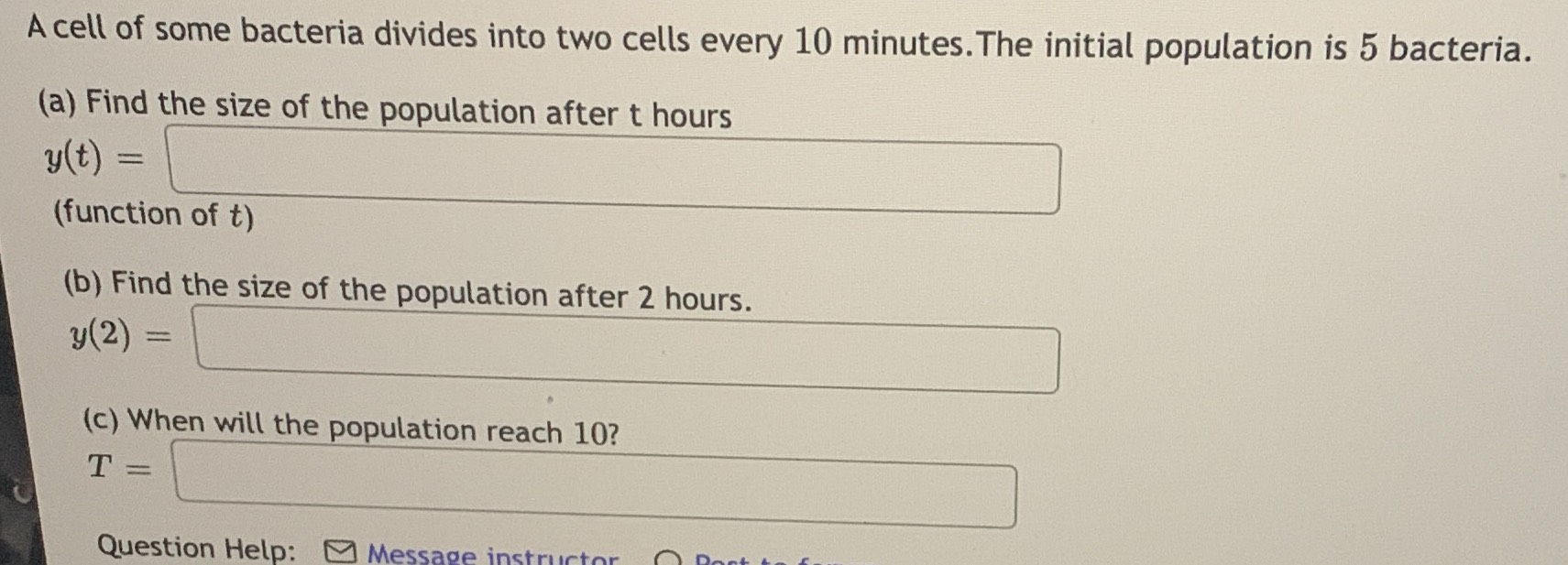### ¿Todavía tienes preguntas de matemáticas?

Pregunte a nuestros tutores expertos
Algebra
PreguntaA cell of some bacteria divides into two cells every $$10$$ minutes. The initial population is $$5$$ bacteria. (a) Find the size of the population after t hours

(b) Find the size of the population after $$2$$ hours.

(c) When will the population reach 10?

a) $$y ( t ) = 5 \times 2 ^ { 6 t }$$